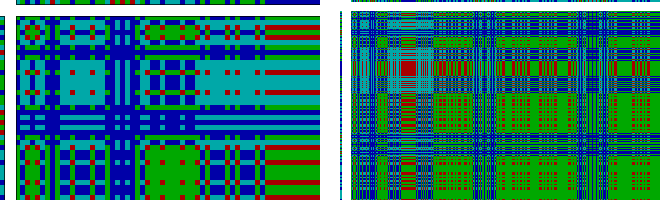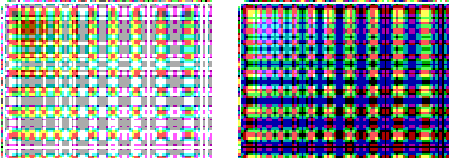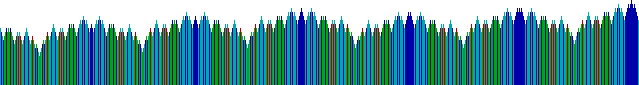'Rug' Enumeration images

We begin with an extremely simple formal system, for which we will construct several different forms of images. The system at issue is propositional logic, made even simpler by restricting it to a single sentence letter p. In order to make things simpler still, we use a single connective: either the Sheffer stroke | , which can be read as NAND, or the dagger, which can be read as NOR. As is well known, either NAND or NOR suffices as a complete base for all Boolean connectives.

Our goal, then, is to construct an image of truth-values for all formulae expressible in terms only of p and | orThe values at issue are merely four, equivalent to p, ~p (p|p or pp) tautologyor contradiction. In Figures 4 through 8 we use the following colors for these values: green for p, blue for ~p, red for tautologies and dark blue for contradictions.

Let us start with a simple 'rug' pattern with an enumeration of all formulae expressible in terms of our single sentence-letter and single connective. For a first enumeration we represent the plan of the rug is laid out schematically as follows (Figure 3)2.Figure 3.

Here formula 1 is p p, formed by a single stroke between the formula heading its row and the formula at the top of its column. That formula, now simply labelled '1', is then placed in the second position along each axis. Formula 2 is formed as a 'slash product' of the formula heading its row and its column -- in the form (row column) -- in the same way. Formula 2 is thus (p|1) or (p|(p|p)). Formula 2 is added as the third formula on each axis. Formula 3 is ((p|p)|p), formula 4 is (p|(p|(p|p))), formula 5 is ((p|p)|(p|p)), formula 6 is ((p|(p|p))|p), and so forth. The pattern continues to generate progressively longer formulae, constituting in the abstract an infinite partial plane extending to the bottom and right and containing all formulae of our simple single- sentence-letter form of propositional calculus.

In Figure 4 the schema is shown in shades of color. Squares correspond directly to the formulae indicated in the schematic sketch above, including formulae along the axes, and are colored in terms of their values: as noted, green = p or equivalent formulae, light blue = p|p or ~p, red represents tautologiesand dark blue represents contradictions. The first graph in Figure 4 is a smaller fragment of the upper left corner of the rug, with the values of formulae indicated on axes as well. The second image in Figure 4 shows a larger section, incorporating the first.Fig. 4
Here a number of systematic features are immediately evident. The first is that the images in Figure 4 are symmetric, reflecting the fact that (x|y) has the same value as (y|x) for any formulae x and y. The 'stripes' in the rug are also obvious, reflecting the fact that both(| x) and (x |) will be tautologous for any x: once any formula on either axis has the value , any formula composed from it with a single stroke will have the value . Closer attention shows that rows in which the value of the formula at the top is will simply reflect the value of the formulae on the column axis, with the same true for columns with the value and the formulae listed along the top.Fig. 5

Figure 5 shows the rug pattern created from the same enumeration of formulae but in which the Sheffer stroke | is replaced with the NOR connective. Side by side, Figures 4 and 5 also serve to make obvious certain relationships between these two connectives: the fact that a contradiction on either side of the stroke gives us a tautology, for example, whereas a tautology on either side of the dagger gives us a contradiction. It is clear that dagger tautologies mirror Sheffer stroke contradictions, with dagger contradictions corresponding to Sheffer tautologies. For simple systems with a single sentence letter, moreover, the values of all contingent formulae in each system are the same. In none of these cases do our images offer genuinely new information regarding the stroke and dagger, of course--all the facts indicated are well known-- though these patterns do make such features vividly evident.Fig. 6
Figures 7 and 8 show a rug pattern using a different enumeration of formulae, following the alternative schematic in Figure 6.Fig. 7 NAND using square enumerationFig. 8 NOR using square enumeration

Nothing, it should be noted, dictates any particular form for enumeration in such a display; nothing dictates the diagonal enumeration of Figure 3 over the square enumeration of figure 6, for example, nor either of these over any of the infinite alternatives. There is therefore an ineliminable arbitrariness to the choice of any particular rug pattern for a formal system. It is also clear, however, that certain properties of patterns--including those noted above--will appear regardless of the pattern of enumeration chosen. Pattern-properties invariant under enumeration can be expected to correspond to deep or basic properties of the system. The rug patterns sketched above are for an extremely simple form of propositional calculus, explicitly restricted to just one sentence letter. Can such an approach be extended to include systems with additional sentence letters as well? Of course. One way of extending the enumeration schemata above so as to include two sentence letters rather than one is simply to begin with the two sentence letters on each axis. In all other regards enumeration can proceed as before (Figure 9):Fig. 9

With two sentence letters, of course, four colors will no longer suffice for values of tautologies, contradictions, and all possible shades of contingency. For a system with both p and q we will require 16 colors in all, corresponding to the sixteen possible truth tables composed of four lines, or equivalently the sixteen binaries composed of four digits. Complete color shade patterns--employing a complete palette of contingencies--are shown for propositional calculus with p and q in Figure 10. These represent NAND and NOR with our initial diagonal enumeration scheme. Corresponding illustrations in terms of our second mode of enumeration appear as Figure 11. It is interesting to note that although a number of the characteristics marked with respect to propositional calculus with a single sentence letter above still hold, one does not: here it is no longer true that contingent values match between NAND and NOR versions. That property, though provable for propositional calculus with a single sentence letter, disappears in richer systems.`NAND                                                                   NOR`

Fig. 10Fig. 11

In both of these illustrations the number of colors at issue becomes even more bewildering in larger sections of the display. In Figures 12 and 13 we have compensated for this difficulty by eliminating all colors for various contingencies in a larger array, leaving only magenta for tautologies and cyan for contradictions. Figure 12 shows NAND and NOR for our first pattern of enumeration; Figure 13 shows NAND and NOR for the second.Fig. 12Fig. 13

In theory any finite number of sentence letters can be added at the beginning of an array in the manner of the enumerations in Figure 9. For n sentence letters, however, the number of colors required to cover all contingencies is 2 raised to 2n colors. At three variables, therefore, we have already hit 28 or 256 contingency colors. At four variables we hit 65,536. In theory the full countable set of sentence letters required for standard propositional calculus might also be introduced along the axes, simply by adding an additional sentence letter at some regular interval (Fig. 14). Because the standard propositional calculus is limited to finite connectives, we would here require countably many contingency colors as well.Fig. 14

Similar representations of formal systems are possible, beyond propositional calculus, for predicate calculus as well. One way of mapping a form of predicate calculus with multiple quantifiers but limited to monadic predicates, for example, would be the following. In a first grid we enumerate all combinations of n-place predicates and variables, giving us Fx, Fy, Fz, ... Gx, gy, Gz., ... These we can think of as a series of propositional functions P1, P2, P3, ..., which can be introduced into a grid for full propositional logic simply by placing them between our progressively introduced sentence letters--p, P1, q, P2, r, P3... -- in an expansion of an enumeration pattern such as that outlined in Figure 14. Quantification over formulae in variables x, y, z... might then be introduced by adding spaced occurrences forx,y,z along just one axis. Here the application of a lone quantifier to formulae in its row could be interpreted as a universal quantification in that variable over that formula. All other intersections would be interpreted as before, in terms for example of the Sheffer stroke (Figure 15). Since existential quantification can be expressed in terms of universal quantification and negation, and the latter can be expressed by the Sheffer stroke in familiar ways, such a schema will include all wffs of predicate calculus involving only monadic predicate letters, together with all corresponding propositional formulae. In assigning values to such a grid, a special 'formula value' would have to be reserved for mere propositional formulae, representing the fact that they fall short of full formal sentences capable of truth values.

This illustration has been limited to monadic predicates simply because the scheme becomes so complicated so quickly even in that case. A representation of the full propositional calculus would demand only the further complication that we include all n-valued Predicate letters paired with n-tuplets of our variables. These can be generated in separate grids first so as to form a single enumeration, then introduced into the main grid in the position of P1, P2, P3... (Figure 15).Fig. 15

On seeing a dog walk on two legs, Abraham Lincoln is reputed to have said, "The amazing thing is not that he does it well but that the thing can be done at all." In something of the same spirit, the purpose of outlining the schema above is simply to show that the thing can be done at all: that the full predicate calculus can be envisaged in the form of a grid of logical values of this kind. In even the simple case of propositional logic with a single sentence letter, however, we remarked on the artificiality introduced by arbitrary choices of enumeration for wffs. In the schema outlined for propositional calculus this artificiality is magnified many times over--by repeated arbitrary choices regarding forms of enumeration within a grid, by choices of how to incorporate different infinite classes of formulae on the axes, and by choices of how to incorporate quantification into the grid. The end product does succeed in showing that the thing can be done. But it should not be expected, we think, to give any particularly perspicuous view of the theorems of the calculus.

If we return for a moment to the simple form of propositional calculus with which we began, restricted to a single sentence letter, it should be clear that either of the enumerations offered above will generate progressively longer wffs. It is not true, of course, that the length of wffs within such an enumeration increases monotonically; formula 10 in our original enumeration is shorter than formula 9, for example. Along the diagonal of either schema, however, formulae do increase in size with each step.Fig. 16

How does such an enumeration look if we graph our formulae sequentially in terms of length with colors assigned for value? The beginning of such a result, using NAND and our first enumeration, is shown in the progressive panels of Figure 16. Colors used are the same as those in the rug patterns above except that tautologies are indicated in white with horizontal cross- hatching so that height will be visible. In the program used for generating this image, one can continue to flip through progressively longer wffs, with no apparent repeat of color patterns.